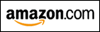INTEGRATION QUIZ #3

NAU STUDENTS:
This exercise gives you new problems to practice—ones requiring the technique of substitution.
Your in-class quiz will cover all the problems from the first two integration quizzes, together with these new problems.

In this exercise, all functions are assumed to have the required properties for a particular situation.
For example, any function in a denominator is assumed to be nonzero, any function inside a logarithm is assumed to be positive, and so on.

## SUBSTITUTION

There's a common theme in mathematics: if you can't solve a problem, try to ‘turn it into’ a problem that you can solve.
Solve the new problem, and then use this answer to solve the original problem.
Substitution is just such a method!

Key idea for substitution: look for something in the integrand whose derivative is also a factor in the integrand, perhaps off by a constant.
This idea is illustrated in the examples below.
This technique is often called ‘$u$-substitution’ since $u$ is a common variable used in the renaming process.
The expression chosen for $\,u\,$ is often something in parentheses, something in an exponent, something in a denominator, or something under a radical.

### EXAMPLE:

In the integral below, note that the derivative of $\,3x^2 - 1\,$ is $\,6x\,$; the variable part of $\,6x\,$ is $\,x\,$.
There is a factor of $\,x\,$ in the integrand! Woo hoo!

Note how we multiply by $\,1\,$ in the form of $\,\frac{6}{6} = 6\cdot\frac{1}{6}\$:
the $\,6\,$ is left inside the integrand (where it becomes part of $\,du\,$) but the $\,\frac{1}{6}\,$ is slid out of the integral.

Here, ‘$\ du = 6x\,dx\$’ can be viewed as a convenient reformulation of $\displaystyle\ \frac{du}{dx} = 6x\$.

Note also that we started with an integral in $\,x\,$, so we must end up with an integral in $\,x\,$.

Let $\,\color{purple}{u = 3x^2-1}\,$, so that $\color{red}{\,du = 6x\ dx\,}$. Then,

$\displaystyle\int x(3x^2 - 1)^7\ dx = \frac{1}{6}\int (\color{purple}{3x^2 - 1})^7\,(\color{red}{6x\,dx}) = \frac{1}{6}\int u^7\ du$

$\displaystyle = \frac{1}{6}\cdot \frac{u^8}{8} + C = \frac{(3x^2-1)^8}{48} + C$

### EXAMPLE:

In the integral below, recall that $\,\sin^5(3x)\,$ is a shorthand for $\,(\sin(3x))^5\,$.
The derivative of $\,\sin(3x)\,$ is $\,3\cos(3x)\,$; the variable part is $\,\cos(3x)\,$, which is a factor in the integrand.

Let $\,u = \sin(3x)\,$, so that $\color{red}{\,du = 3\cos(3x)\ dx\,}$. Then,

$\displaystyle\int \sin^5(3x)\cos(3x)\ dx = \frac{1}{3}\int (\color{purple}{\sin(3x)})^5 (\color{red}{3\cos 3x\ dx}) = \frac{1}{3}\int u^5\ du$

$\displaystyle = \frac{1}{3}\cdot \frac{u^6}{6} + C = \frac{\sin^6(3x)}{18} + C$
Master the ideas from this section
by practicing the exercise at the bottom of this page.

On this exercise, you will not key in your answer.
However, you can check to see if your answer is correct.Is this web exercise helping you? I'm spending many hours creating it. Please help me to help you... just watch my 15-second video to see how easy it is to support my work, at absolutely no cost to you! (Really!) Thank you!